Screenshots

•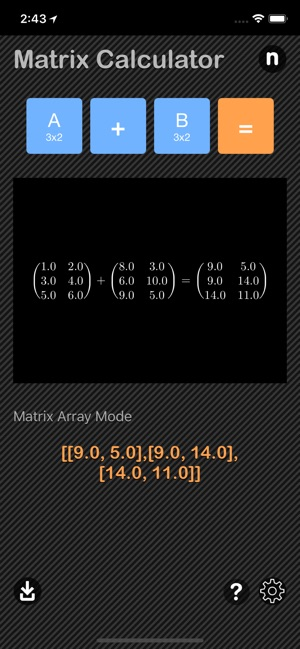•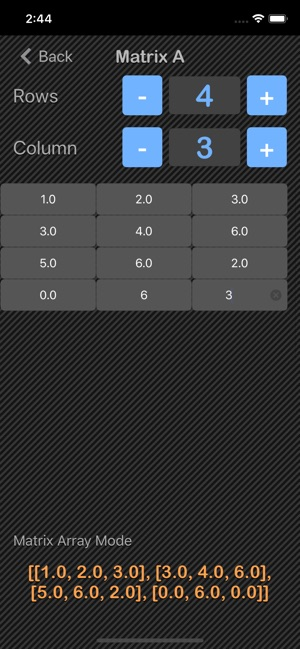•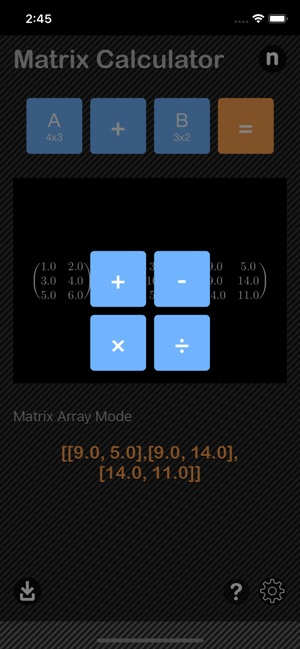•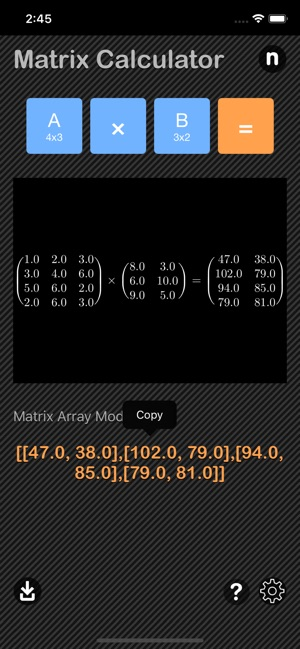•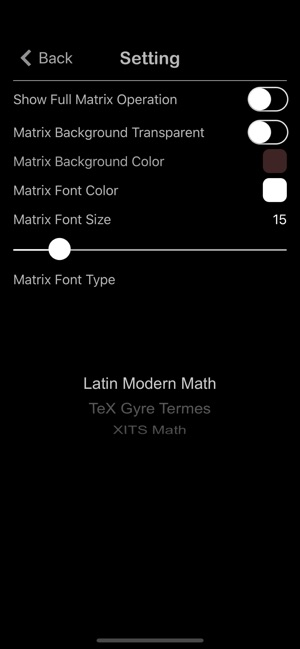•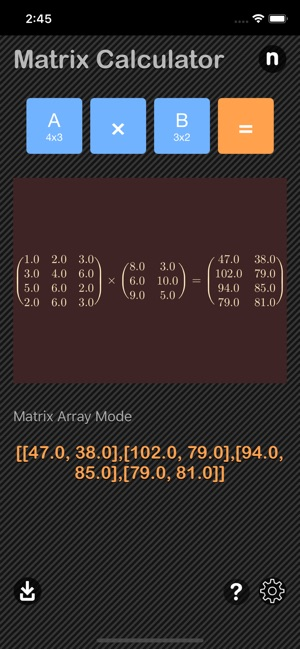Description

Matrix Calculator are math calculator to solve matrix operations and functions fast and easy.

Features:
- Support matrix addition, subtracting, multiplication and dividing
- Generate Matrix in Latex mode and save-able to cameraroll
- Matrix in array mode are copy-able
- Matrix reference included

In mathematics, a matrix (plural: matrices) is a rectangular array of numbers, symbols, or expressions, arranged in rows and columns.

To add two matrices or Subtracting
A matrix A with 3 rows and 5 columns can be added to another matrix B of 3 rows and 5 columns.
The two matrices must be the same size, i.e. the rows must match in size, and the columns must match in size.

To multiply an m×n matrix by an n×p matrix, the ns must be the same,
and the result is an m×p matrix.
mxn x nxp = mxp

To divide two matrices
A/B = A × (1/B) = A × B⁻¹

*This is a universal app that work for both iPhone and iPad.

Thanks for your support and do visit nitrio.com for more apps for your iOS devices.

Information

Seller
Tan Chia Ling
Size
21.6 MB
Category
Utilities
Compatibility

Requires iOS 8.0 or later. Compatible with iPhone, iPad, and iPod touch.

Languages

English

Age Rating
Rated 4+
•